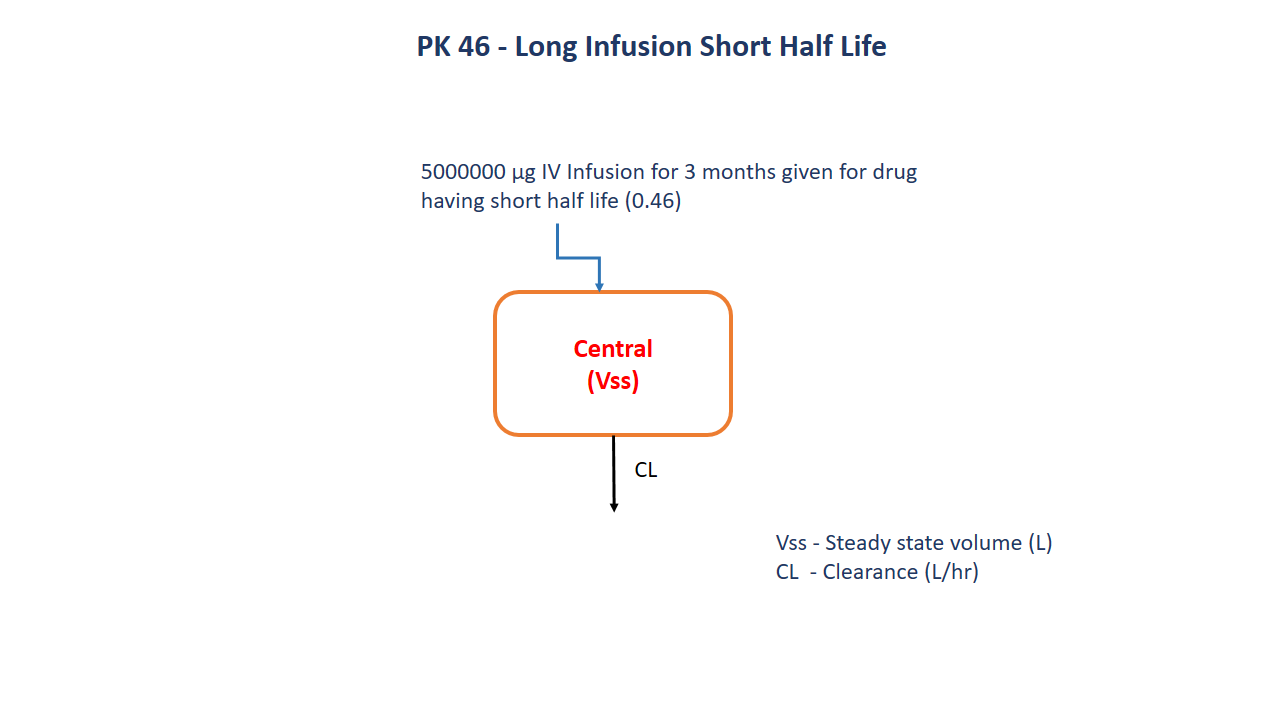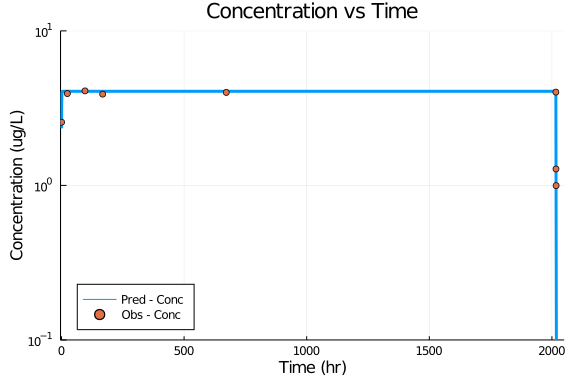# Exercise 46 - Long Infusion Short Half Life

### Background

• Structural model - Long infusion short half life one compartment model

• Route of administration - IV infusion

• Dosage Regimen - 500000 ug

• Number of Subjects - 1### Learning Outcome

This exercise deals with the drug having short half life.

### Objectives

To build a model for drug having short half life given as a very long infusion up to 3 months.

### Libraries

call the "necessary" libraries to get started.

using Pumas
using Plots
using CSV
using StatsPlots
using Random


### Model

To build a one compartment model for a drug having short half life given a long infusion for 3 months.

pk_46         = @model begin
@param begin
tvvss   ∈ RealDomain(lower=0)
tvcl    ∈ RealDomain(lower=0)
Ω       ∈ PDiagDomain(2)
end

@random begin
η       ~ MvNormal(Ω)
end

@pre begin
Vss     = tvvss*exp(η)
Cl      = tvcl*exp(η)
end

@dynamics begin
Central' =  - (Cl/Vss)*Central
end

@derived begin
cp       = @. Central/Vss
end
end

PumasModel
Random effects: η
Covariates:
Dynamical variables: Central
Derived: cp, dv
Observed: cp, dv


### Parameters

Parameters provided for simulation are as below. tv represents the typical value for parameters.

• Vss - Steady state volume (L)

• CL - Clearance (L/hr)

param = ( tvvss  = 35.6,
tvcl   = 61,
Ω      = Diagonal([0.0,0.0]),

(tvvss = 35.6, tvcl = 61, Ω = [0.0 0.0; 0.0 0.0], σ_add = 0.196337)


### DosageRegimen

A dose of 500000 ug was given as an Intravenous Infusion to a single subject for over 3 months.

ev1  = DosageRegimen(500000, time=0, cmt=1, duration=2016)
sub1 = Subject(id=1, events=ev1)

Subject
ID: 1
Events: 2


### Simulation

Simulate the data after the administration of Infusion

Random.seed!(123)
sim_sub1 = simobs(pk_46,sub1,param,obstimes=0.5:0.1:2100)
df1      = DataFrame(sim_sub1)


### Dataframe & Plot

Use the dataframe for plotting

df1_dv = filter(x -> x.time in [0.5,24,96,168,672,2016,2016.5,2017,2018], df1)

@df df1 plot(:time, :cp, yaxis=:log, legend=:bottomleft,
title="Concentration vs Time", label="Pred - Conc",
xlabel="Time (hr)", ylabel="Concentration (ug/L)", linewidth=3,
yticks=[0.1,1,10], ylims=(0.1,10), xticks=[0,500,1000,1500,2000], xlims=(-4,2050))
@df df1_dv scatter!(:time, :dv, label="Obs - Conc")